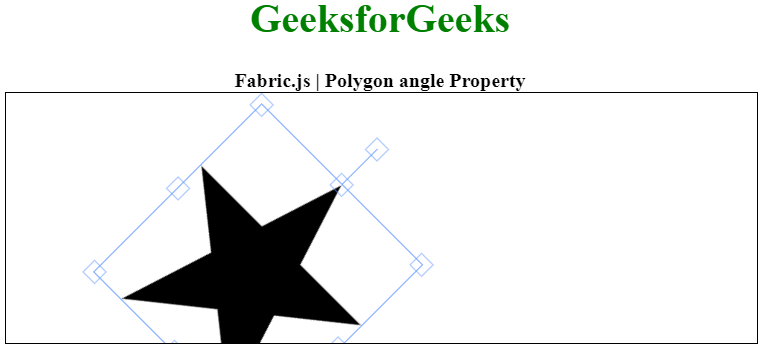Related Articles

# Fabric.js Polygon angle Property

• Last Updated : 18 Jan, 2021

In this article, we are going to see how to set the angle of a canvas Polygon using FabricJS. The canvas Polygon means the Polygon is movable and can be stretched according to requirement. Further, the polygon can be customized when it comes to initial stroke color, height, width, fill color, or stroke width.

To make this possible we are going to use a JavaScript library called FabricJS. After importing the library, we will create a canvas block in the body tag which will contain the polygon. After this, we will initialize instances of Canvas and Polygon provided by FabricJS and enable the angle of the canvas Polygon using angle property and render the Polygon on the Canvas as given below.

Syntax:

```fabric.Polygon([
{ x: pixel, y: pixel },
{ x: pixel, y: pixel },
{ x: pixel, y: pixel},
{ x: pixel, y: pixel},
{ x: pixel, y: pixel }],
{
angle: number
});```

Parameters: This property accepts a single parameter as mentioned above and described below:

• angle: This parameter defines the angle of the polygon in degrees.

The below example illustrates the Fabric.JS Polygon angle property in JavaScript:

Example: In this example, we have set the angle property of the polygon to 45 degrees.

## HTML

 ` ``<``html``> `` ` `<``head``> ``    ````    ``<``script` `src``= ``"https://cdnjs.cloudflare.com/ajax/libs/fabric.js/3.6.2/fabric.min.js"``> ``    `` `` `` ` `<``body``> ``    ``<``div` `style``=``"text-align: center;width: 600px;"``> ``        ``<``h1` `style``=``"color: green;"``> ``            ``GeeksforGeeks ``        `` ``        ``<``b``> ``            ``Fabric.js | Polygon angle Property ``        `` ``    `` `` ` `    ``<``canvas` `id``=``"canvas"``            ``width``=``"600"``            ``height``=``"200"``            ``style``=``"border:1px solid #000000;"``> ``    `` `` ` `    ``<``script``> ``        ``// Initiate a Canvas instance ``        ``var canvas = new fabric.Canvas("canvas"); `` ` `        ``// Initiate a polygon instance ``        ``var polygon = new fabric.Polygon([ ``        ``{ x: 295, y: 10 }, ``        ``{ x: 235, y: 198 }, ``        ``{ x: 385, y: 78}, ``        ``{ x: 205, y: 78}, ``        ``{ x: 355, y: 198 }], { ``            ``angle: 45 ``        ``}); `` ` `        ``// Render the polygon in canvas ``        ``canvas.add(polygon); ``    `` `` `` ` ``

Output:My Personal Notes arrow_drop_up